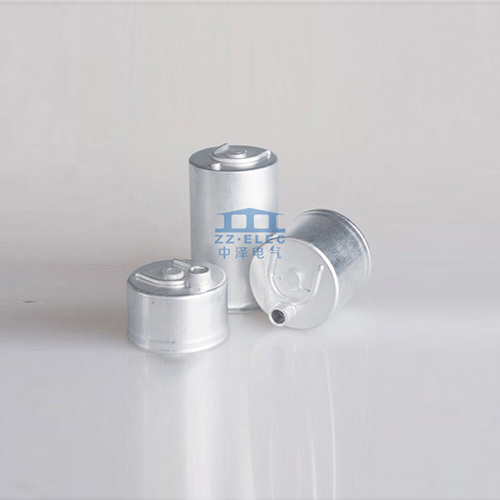# What are the error levels of capacitors?

Update:09,Oct,2020
Summary: Representation methods include direct standard method, color circle method and ...
Representation methods include direct standard method, color circle method and digital method. The color circle method and the meaning of the color circle are the same as resistors. The digital method is generally represented by three digits. Counting from the left, the first and second digits are the one and two digits of the capacity, and the third digit represents the first two digits and multiplied by the power of 10. The unit of capacity is PF, usually with a letter after three digits to indicate the error. Capacitor aluminum shell manufacturers about capacitance:Electrolytic capacitor: small size, large capacity, but it has polarity, the polarity can not be connected wrongly, and the capacity value is unstable, the leakage is large, and it is easy to age, even if it is not used for a long time, it is easy to deteriorate and the capacity will decrease. When measuring the electrolytic capacitor with the resistance profile of a multimeter, after the pointer of the meter swings to the value, it should return to the starting point or close to the starting point; the greater the swing of the pointer, the larger the capacitance, and the closer the pointer is to the starting point, the capacitance The smaller the leakage, the larger the insulation resistance; if the pointer does not swing or does not return after swinging, it means that the capacitor has been broken or short-circuited.
Common faults of capacitors are mainly open circuit, short circuit, capacity loss and leakage. Large-capacity capacitors can be found with a multimeter in the same way as "electrolytic capacitors". In addition to short-circuit and serious leakage of small-capacity capacitors, other faults are not easy to check with ordinary multimeters. Some mechanical multimeters have a file for measuring capacitance, but an external power supply is required (see the instruction manual of the multimeter for usage), and some digital multimeters (including digital capacitance meters) have a file for directly measuring capacitance.
Pay attention to the capacitance value and withstand voltage value of the component when replacing the capacitor.
When two capacitors are connected in series: the total capacity C=(C1×C2)/(C1+C2); the withstand voltage after the series connection is: if the capacity of each capacitor in the series is equal, the voltage it bears is also equal; if the capacity is not equal, then The larger the capacity, the smaller the voltage it bears, and the smaller the capacity, the higher the voltage it bears. (Because the charging current of each capacitor is equal when connected in series, the sum of its voltage drops is equal to the total voltage).
When capacitors are connected in parallel: total capacity C=C1+C2+C3+……; the voltage that each capacitor bears in parallel is the circuit voltage.